# Detect Issues During Deep Neural Network Training

This example shows how to automatically detect issues while training a deep neural network.

When you train networks for deep learning, it is often useful to monitor the training progress. In this example, use a `trainingProgressMonitor` object to check if your network is overfitting during training.

Load the digits data as an image datastore using the `imageDatastore` function and specify the folder containing the image data.

```dataFolder = fullfile(toolboxdir("nnet"),"nndemos","nndatasets","DigitDataset"); imds = imageDatastore(dataFolder, ... IncludeSubfolders=true, .... LabelSource="foldernames");```

Choose 70% of the data for training and 30% for validation. To demonstrate overfitting, this example does not randomize the data split.

```trainingProportion = 0.7; [imdsTrain,imdsValidation] = splitEachLabel(imds,trainingProportion);```

The network used in this example requires input images of size 28-by-28-by-1. To automatically resize the training and validation images, use an augmented image datastore.

```inputSize = [28 28 1]; augimdsTrain = augmentedImageDatastore(inputSize(1:2),imdsTrain); augimdsValidation = augmentedImageDatastore(inputSize(1:2),imdsValidation);```

Determine the number of classes in the training data.

```classes = categories(imdsTrain.Labels); numClasses = numel(classes);```

### Define Network

Define the network for image classification.

• For image input, specify an image input layer with input size matching the training data.

• Do not normalize the image input, set the `Normalization` option of the input layer to `"none"`.

• Specify three convolution-batchnorm-ReLU blocks.

• Pad the input to the convolution layers such that the output has the same size by setting the `Padding` option to `"same"`.

• For the first convolution layer, specify 20 filters of size 5. For the remaining convolution layers, specify 20 filters of size 3.

• For classification, specify a fully connected layer with size matching the number of classes

• To map the output to probabilities, include a softmax layer.

When training a network using a custom training loop, do not include an output layer.

```layers = [ imageInputLayer(inputSize,Normalization="none") convolution2dLayer(5,20,Padding="same") batchNormalizationLayer reluLayer convolution2dLayer(3,20,Padding="same") batchNormalizationLayer reluLayer convolution2dLayer(3,20,Padding="same") batchNormalizationLayer reluLayer fullyConnectedLayer(numClasses) softmaxLayer];```

Create a `dlnetwork` object from the layer array.

`net = dlnetwork(layers);`

### Define Model Loss Function

Training a deep neural network is an optimization task. By considering a neural network as a function $\mathit{f}\left(\mathit{X};\Theta \right)$, where $\mathit{X}$ is the network input and $\Theta$ is the set of learnable parameters, you can optimize $\Theta$ so that it minimizes some loss value based on the training data. For example, optimize the learnable parameters $\Theta$ such that for a given inputs $\mathit{X}$ with a corresponding targets $\mathit{T}$, they minimize the error between the predictions $\mathit{Y}=\mathit{f}\left(\mathit{X},\Theta \right)$ and $\mathit{T}$.

Create the function `modelLoss`, listed in the Model Loss Function section of the example, that takes as input the `dlnetwork` object, a mini-batch of input data with corresponding targets, and returns the loss, the gradients of the loss with respect to the learnable parameters, and the network state.

### Specify Training Options

Train for five epochs with a mini-batch size of 128. Specify the options for SGDM optimization. Specify an initial learn rate of 0.01 and momentum 0.9. Try experimenting with different values for the number of epochs and the learn rate.

```numEpochs =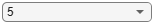5; miniBatchSize = 128; learnRate =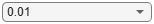0.01; momentum = 0.9;```

Evaluate the model on the validation data every 10 iterations.

`validationFrequency = 10;`

### Define Overfitting Check Function

Create the function `checkForOverfitting`, listed in the Overfitting Check Function section of the example. This function takes metric data containing the training and validation accuracy, and determines if the network is overfitting by checking if the ratio of validation accuracy to training accuracy is less than the threshold specified.

`$\mathrm{Overfitting}\text{\hspace{0.17em}}\mathrm{Ratio}=\frac{\mathrm{Validation}\text{\hspace{0.17em}}\mathrm{Accuracy}}{\mathrm{Training}\text{\hspace{0.17em}}\mathrm{Accuracy}}$`

If the overfitting ratio is less than the overfitting threshold, then the network is overfitting. Specify an overfitting threshold of 0.9.

`overFittingThreshold =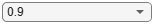0.9;`

### Train Model

Create a `minibatchqueue` object that processes and manages mini-batches of images during training. For each mini-batch:

• Use the custom mini-batch preprocessing function `preprocessMiniBatch` (defined at the end of this example) to convert the labels to one-hot encoded variables.

• Format the image data with the dimension labels `"SSCB"` (spatial, spatial, channel, batch). By default, the `minibatchqueue` object converts the data to `dlarray` objects with underlying type `single`. Do not format the class labels.

• Train on a GPU if one is available. By default, the `minibatchqueue` object converts each output to a `gpuArray` if a GPU is available. Using a GPU requires Parallel Computing Toolbox™ and a supported GPU device. For information on supported devices, see GPU Computing Requirements (Parallel Computing Toolbox).

```mbq = minibatchqueue(augimdsTrain, ... MiniBatchSize=miniBatchSize, ... MiniBatchFcn=@preprocessMiniBatch, ... MiniBatchFormat=["SSCB" ""]);```

Initialize the velocity parameter for the SGDM solver.

`velocity = [];`

Find the total number of iterations.

`totalIterations = numEpochs*ceil(augimdsTrain.NumObservations/miniBatchSize);`

Initialize the `TrainingProgressMonitor` object. Because the timer starts when you create the monitor object, make sure that you create the object close to the training loop.

```monitor = trainingProgressMonitor(... Metrics=["TrainingLoss","ValidationLoss","TrainingAccuracy","ValidationAccuracy"], ... Info=["Epoch","MaxEpochs"], ... XLabel="Iteration", ... Status="Training..."); groupSubPlot(monitor,Accuracy=["TrainingAccuracy","ValidationAccuracy"]); groupSubPlot(monitor,Loss=["TrainingLoss","ValidationLoss"]); updateInfo(monitor,MaxEpochs=numEpochs);```

Initialize the monitor with the training check information using the `showCheckOnPlot` function defined at the end of this example. The `showCheckOnPlot` function creates a `struct` with information that you can use to update the overfitting check during training.

`trainingCheck = showCheckOnPlot(monitor,overFittingThreshold);`

Train the network using a custom training loop. For each epoch, shuffle the data and loop over mini-batches of data. For each mini-batch:

• Evaluate the model loss, gradients, and state using the `dlfeval` and `modelLoss` functions and update the network state.

• Update the network parameters using the `sgdmupdate` function.

• Display the training progress.

• Check for overfitting every validation iteration.

• Stop if the `Stop` property is true. The `Stop` property value of the `TrainingProgressMonitor` object changes to true when you click the Stop button in the Training Progress window.

```iteration = 0; epoch = 0; % Loop over epochs. while epoch < numEpochs && ~monitor.Stop epoch = epoch + 1; % Shuffle data. shuffle(mbq); % Loop over mini-batches. while hasdata(mbq) && ~monitor.Stop iteration = iteration + 1; % Read mini-batch of data. [X,T] = next(mbq); % Evaluate the model loss, gradients, and state using dlfeval and the % modelLoss function and update the network state. [loss,gradients,state] = dlfeval(@modelLoss,net,X,T); net.State = state; % Compute the training accuracy. Y = predict(net,X); accuracyTrain = 100*mean(onehotdecode(Y,classes,1) == onehotdecode(T,classes,1)); % Update the network parameters using the SGDM optimizer. [net,velocity] = sgdmupdate(net,gradients,velocity,learnRate,momentum); % Display the training progress. recordMetrics(monitor,iteration, ... TrainingLoss=loss, ... TrainingAccuracy=accuracyTrain); % Calculate the validation accuracy. if iteration == 1 || mod(iteration,validationFrequency) == 0 [lossVal,accuracyVal] = calculateValidationMetrics(net,augimdsValidation,miniBatchSize,classes); recordMetrics(monitor,iteration, ... ValidationAccuracy=accuracyVal, ... ValidationLoss=lossVal); % Check if the model is overfitting. trainingCheck = updateTrainingChecks(monitor,trainingCheck); end % Update the training progress bar. monitor.Progress = 100*iteration/totalIterations; end % Update the epoch on the training progress monitor. updateInfo(monitor,Epoch=epoch); end % Set the final status on the monitor. if monitor.Stop monitor.Status = "Training Stopped"; else monitor.Status = "Training Complete"; end```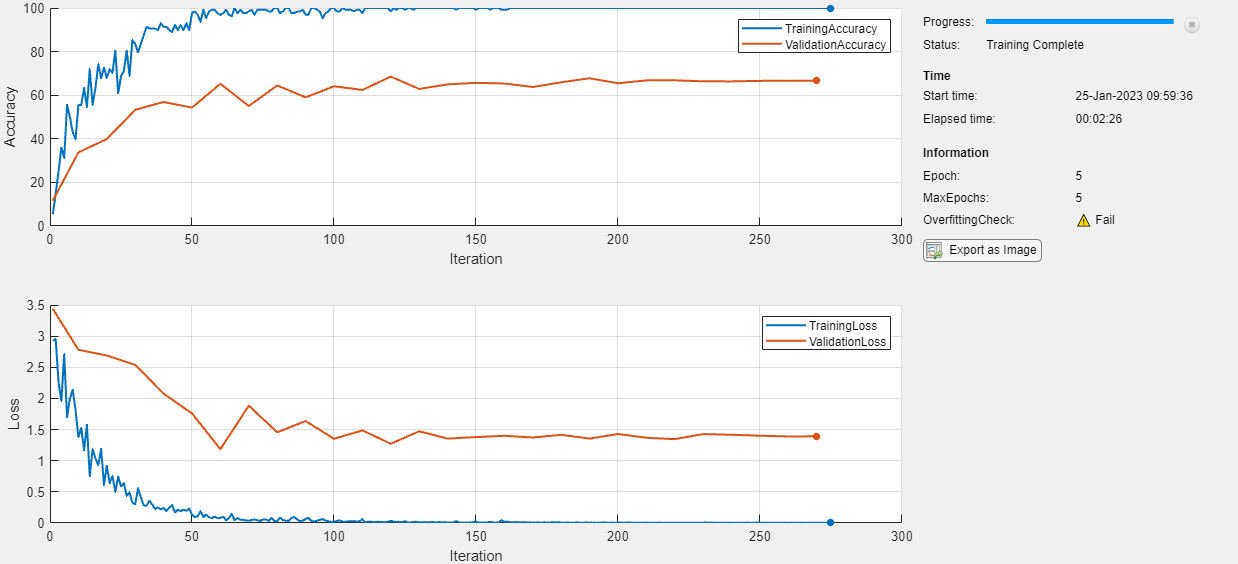### Check for Overfitting

Find the last value of the overfitting check for the model.

`trainingCheck.LastValue`
```ans = 0 ```

A `LastValue` value of 0 means that the model is overfitting. To prevent overfitting, try one or more of the following:

• Randomize the data

• Use data augmentation

• Use dropout layers

• Increase the regularization factor.

In this example, to prevent overfitting, randomize your data before training. To randomize the data, specify `"randomized"` when using the `splitEachLabel` function.

`[imdsTrain,imdsValidation] = splitEachLabel(imds,trainingProportion,"randomized");`

If you train the model again with the data randomized, then the model passes the overfitting check.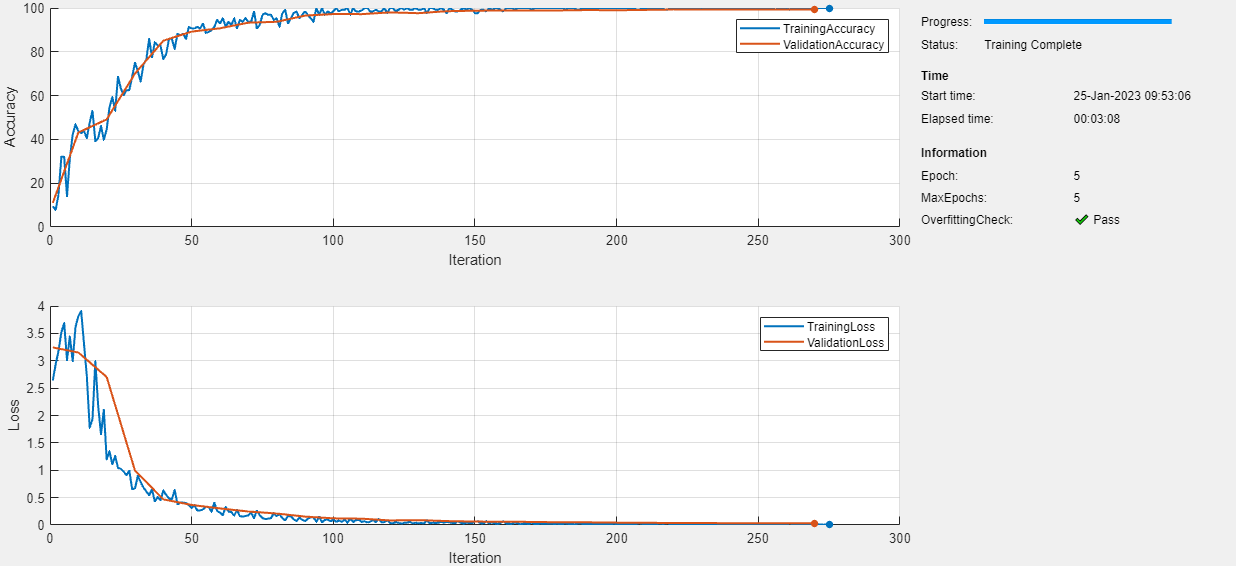### Supporting Functions

#### Model Loss Function

The `modelLoss` function takes a `dlnetwork` object `net`, a mini-batch of input data `X` with corresponding targets `T` and returns the loss, the gradients of the loss with respect to the learnable parameters in `net`, and the network state. To compute the gradients automatically, use the `dlgradient` function.

```function [loss,gradients,state] = modelLoss(net,X,T) % Forward data through network. [Y,state] = forward(net,X); % Calculate cross-entropy loss. loss = crossentropy(Y,T); % Calculate gradients of loss with respect to learnable parameters. gradients = dlgradient(loss,net.Learnables); end```

#### Validation Metrics Function

The `calculateValidationMetrics` function takes a network, `augmentedImageDatastore` object containing the validation data, mini-batch size, and classes, and returns the loss and accuracy for the validation data.

```function [loss,accuracy] = calculateValidationMetrics(net,augvalDatastore,miniBatchSize,classes) % Pass the validation data through the network in batches. mbq = minibatchqueue(augvalDatastore, ... MiniBatchSize=miniBatchSize, ... MiniBatchFcn=@preprocessMiniBatch, ... MiniBatchFormat=["SSCB",""]); T = []; Y = []; % Loop over mini-batches. while hasdata(mbq) [X,batchT] = next(mbq); % Pass the data through the network. batchY = predict(net,X); % Append to the output. Y = [Y,batchY]; T = [T,batchT]; end % Calculate the cross-entropy loss. loss = crossentropy(Y,T); % Compute the accuracy. accuracy = 100*mean(onehotdecode(Y,classes,1) == onehotdecode(T,classes,1)); end```

#### Mini-Batch Preprocessing Function

The `preprocessMiniBatch` function preprocesses a mini-batch of predictors and labels using the following steps:

1. Preprocess the images using the `preprocessMiniBatchPredictors` function.

2. Extract the label data from the incoming cell array and concatenate into a categorical array along the second dimension.

3. One-hot encode the categorical labels into numeric arrays. Encoding into the first dimension produces an encoded array that matches the shape of the network output.

```function [X,T] = preprocessMiniBatch(dataX,dataT) % Preprocess predictors. X = preprocessMiniBatchPredictors(dataX); % Extract label data from cell and concatenate. T = cat(2,dataT{1:end}); % One-hot encode labels. T = onehotencode(T,1); end```

#### Mini-Batch Predictors Preprocessing Function

The `preprocessMiniBatchPredictors` function preprocesses a mini-batch of predictors by extracting the image data from the input cell array and concatenating it into a numeric array. For grayscale input, concatenating over the fourth dimension adds a third dimension to each image, to use as a singleton channel dimension.

```function X = preprocessMiniBatchPredictors(dataX) % Concatenate. X = cat(4,dataX{1:end}); end```

#### Initialize Training Check

The `showCheckOnPlot` function creates a `struct` with information that you can use to update the overfitting check during training.

• `Name` — Display name of the check in the training progress monitor window.

• `CheckFunction` — Function handle to use to check for issues. For more information, see the `checkForOverfitting` function.

The function adds the check to the `TrainingProgressMonitor` object and displays the result in the Training Progress window. You can add more checks to the `struct` by defining more check functions and adding them to the `struct`.

```function trainingCheck = showCheckOnPlot(monitor,threshold) trainingCheck = struct("Name","OverfittingCheck", ... "CheckFunction",@(x)checkForOverfitting(x,threshold)); % Create an info item on the training progress monitor for the % training check. monitor.Info = [monitor.Info trainingCheck.Name]; updateInfo(monitor,trainingCheck.Name,"❓ Unknown"); end```

#### Update Training Check

The `updateTrainingChecks` function takes as input a `TrainingProgressMonitor` object and a training check. The function verifies if the check passes by calling `CheckFunction` and updates `LastValue` with the result. If the check passes, then the check value is 1 and no issue was detected. If the check fails, then the check value is 0 and an issue was detected. The function updates the training progress monitor with the latest results.

```function trainingCheck = updateTrainingChecks(monitor,trainingCheck) % Update the training check. check = trainingCheck.CheckFunction(monitor.MetricData); if check == 0 % Check failed. updateInfo(monitor,trainingCheck.Name,"⚠️ Fail"); trainingCheck.LastValue = 0; elseif check == 1 % Check passed. updateInfo(monitor,trainingCheck.Name,"✔️ Pass") trainingCheck.LastValue = 1; else % Check unverified. Use the existing value % in the Info field. end end```

#### Overfitting Check Function

This `checkForOverfitting` function takes metric data containing the training and validation accuracy and determines if the model is not overfitting by checking if the ratio of validation accuracy to training accuracy is greater than or equal to the threshold given.

```function result = checkForOverfitting(metricData,threshold) % Set the number of training points to average the check across. n = 10; % If there is no value for one or other of the training or validation % accuracies, then return unknown. trainingAccData = metricData.TrainingAccuracy; if size(trainingAccData,1) < n result = -1; else % Check that the ratio of the last validation accuracy % to the average of the last 'n' training accuracy is % greater than the chosen threshold. avgTrainAcc = mean(trainingAccData(end-n+1:end)); validationAccData = metricData.ValidationAccuracy; lastValidationAccPoint = validationAccData(end,:); result = lastValidationAccPoint(2)/avgTrainAcc >= threshold; end end```